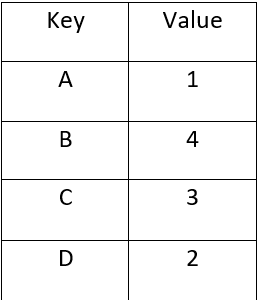# Java Program to Implement Control Table in Java

• Last Updated : 20 Nov, 2020

Control tables will be tables that control the control stream or have a significant influence on program control. There are no unbending guidelines about the structure or substance of a control table—its passing trait is its capacity to coordinate the control stream somehow or another through “execution” by a processor or mediator. The plan of such tables is now and again alluded to as a table-driven plan.

In maybe its least difficult execution, a control table may here and there be a one-dimensional table for legitimately deciphering a crude information incentive to a comparing subroutine balance, record, or pointer utilizing the crude information esteem either straightforwardly as the list to the exhibit, or by playing out some fundamental number-crunching on the information previously. Control tables lessen the requirement for programming comparable structures or program explanations again and again. The two-dimensional nature of most tables makes them simpler to view and refresh than the one-dimensional nature of program code.

Beneath Java Program exhibits the usage of the control Table for changing the ASCII Codes. We have utilized the populateTable() Method, which utilizes HashMap to Store the new ASCII Codes. It doesn’t need any contentions.Control Table to store new ASCII Codes.

## Java

 `// Java Program to Implement Control Table in Java`` ` `import` `java.util.*;`` ` `public` `class` `ControlTables {``    ``private` `Map controlTable;`` ` `    ``public` `ControlTables()``    ``{``        ``// ControlTables Constructor``        ``controlTable = ``new` `HashMap();``        ``populateTable();``    ``}`` ` `    ``public` `int``[] controlTable(``int``[] asciiCodes)``    ``{``        ``// Returns the Index of the Desired ASCII Code``        ``// present in the Map controlTables``        ``int``[] index = ``new` `int``[asciiCodes.length];``        ``for` `(``int` `val = ``0``; val < asciiCodes.length; val++) {``            ``index[val] = controlTable.get(``                ``Integer.toHexString(asciiCodes[val]));``        ``}``        ``return` `index;``    ``}`` ` `    ``private` `void` `populateTable()``    ``{``        ``// Method inserts the new ASCII codes to HashMap``        ``// controlTable`` ` `        ``/*The Java.lang.Integer.toHexString() is a built-in``         ``* function in Java which returns a string``         ``* representation of the integer argument as an``         ``* unsigned integer in base 16. The function accepts``         ``* a single parameter as an argument in Integer``         ``* data-type.``         ``*/``        ``controlTable.put(Integer.toHexString(``65``), ``01``);``        ``controlTable.put(Integer.toHexString(``66``), ``04``);``        ``controlTable.put(Integer.toHexString(``67``), ``03``);``        ``controlTable.put(Integer.toHexString(``68``), ``02``);``    ``}``    ``public` `static` `void` `main(String[] args)``    ``{`` ` `        ``int``[] asciiCodes = ``new` `int``[``4``];``        ``int``[] tableOutput;``        ``asciiCodes[``0``] = (``int``)``'A'``;``        ``asciiCodes[``1``] = (``int``)``'B'``;``        ``asciiCodes[``2``] = (``int``)``'C'``;``        ``asciiCodes[``3``] = (``int``)``'D'``;`` ` `        ``ControlTables controlTable = ``new` `ControlTables();``        ``tableOutput = controlTable.controlTable(asciiCodes);`` ` `        ``System.out.println(``"Input values "``);``        ``System.out.print(``"( "``);``        ``for` `(``int` `i = ``0``; i < asciiCodes.length; i++) {``            ``System.out.print((``char``)asciiCodes[i] + ``" "``);``        ``}``        ``System.out.println(``")"``);`` ` `        ``System.out.println(``"New Index from Control table"``);``        ``System.out.print(``"( "``);``        ``for` `(``int` `i = ``0``; i < tableOutput.length; i++) {``            ``System.out.print(tableOutput[i] + ``" "``);``        ``}``        ``System.out.print(``")"``);``    ``}``}`

Output

```Input values
( A B C D )
New Index from Control table
( 1 4 3 2 )```

My Personal Notes arrow_drop_up